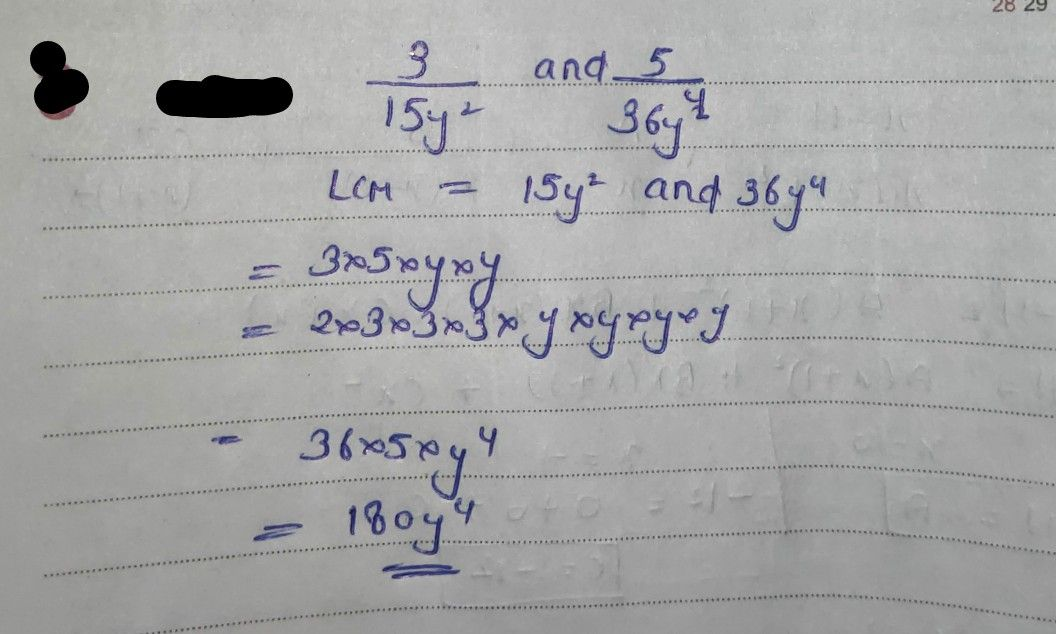Symbol
ProblemWhat I Know $oirecbons$ Choose the correct answer. Write your answer on a separate sheet 1. Give the Least Common Denominator $\left(lCD\right)ot\dfrac {3} {15y^{2}}and$ A. 36y2 $\dfrac {5} {36y^{4}}$ $90y^{2}$ B. 36y* C. D. $180y^{4}$ 2. Find the $eLcoot\dfrac {7} {8-2a}and\dfrac {2} {4-a}$ $A\left(4-a\right)$ $B.2\left(4-a\right)$ $c.\left(a^{2}+64\right)$ $D.\left(64-a^{2}\right)$ 3. Give the sum of $A\dfrac {a^{2}} {b2}$ $B\dfrac {a^{2}} {b}$ D. 4. Find simplified form of $\dfrac {2x} {2}+\dfrac {x} {3}$ $A\dfrac {4x} {2}$ $B.\dfrac {5x} {3}$ D. 5. Perform the indicated operation -. A B. *** * c X-6 D. -10 6. Look for the sum $αt\dfrac {3x-5} {2}+\dfrac {x+3} {2}$ $Ax-1$ $B.x-2$ $C.x-3$ $D.x-4$ 7. Given as one addend of the sum Bx-7 find the other addend. $n\dfrac {x+1} {3}$ $\dfrac {7x-4} {3}$ $\dfrac {7x-6} {3}$ C. B. D. 77x3 -2-18 0 8. Find the sum of A. 13x-2 B. $\dfrac {n} {2x\left(x-2\right)}$ $\dfrac {ax-10} {2x\left(x-2\right)}$ C. $2x\left(x-2$ 13x-6 D. $2x\left(x-2$
7th-9th grade
Other
Search count: 125
SolutionQanda teacher - Askhina